Updating search results...

# 706 Results

View
Selected filters:
• Algebra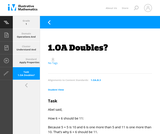Unrestricted Use
CC BY
Rating
0.0 stars

This is a task from the Illustrative Mathematics website that is one part of a complete illustration of the standard to which it is aligned. Each task has at least one solution and some commentary that addresses important aspects of the task and its potential use.

Subject:
Algebra
Material Type:
Activity/Lab
Provider:
Illustrative Mathematics
Provider Set:
Illustrative Mathematics
Author:
Illustrative Mathematics
08/06/2015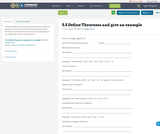Unrestricted Use
CC BY
Rating
0.0 stars

College Algebra
A pre-lecture worksheet for students to preview section 5.5 and find the descriptions for the theorems covered in this section. Also having them try an example for each of the theorems.

Subject:
Algebra
Material Type:
Homework/Assignment
Author:
Sandy Porter
04/19/2019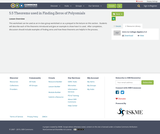Unrestricted Use
CC BY
Rating
0.0 stars

This worksheet can be used as an in-class group worksheet or as a prequel to the lecture on this section.  Students will describe each of the theorems introduced and give an example to show how it is used.  After completion, discussion should include examples of finding zeros and how these theorems are helpful in the process.

Subject:
Algebra
Material Type:
Lesson Plan
Author:
Sandy Porter
04/18/2019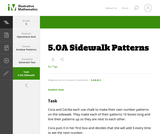Unrestricted Use
CC BY
Rating
0.0 stars

This is a task from the Illustrative Mathematics website that is one part of a complete illustration of the standard to which it is aligned. Each task has at least one solution and some commentary that addresses important aspects of the task and its potential use.

Subject:
Algebra
Material Type:
Activity/Lab
Provider:
Illustrative Mathematics
Provider Set:
Illustrative Mathematics
Author:
Illustrative Mathematics
08/06/2015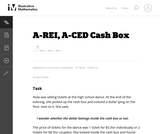Unrestricted Use
CC BY
Rating
0.0 stars

The given solutions for this task involve the creation and solving of a system of two equations and two unknowns, with the caveat that the context of the problem implies that we are interested only in non-negative integer solutions. Indeed, in the first solution, we must also restrict our attention to the case that one of the variables is further even.

Subject:
Mathematics
Algebra
Material Type:
Activity/Lab
Provider:
Illustrative Mathematics
Provider Set:
Illustrative Mathematics
Author:
Illustrative Mathematics
01/18/2013Unrestricted Use
CC BY
Rating
0.0 stars

This task examines the ways in which the plane can be covered by regular polygons in a very strict arrangement called a regular tessellation. These tessellations are studied here using algebra, which enters the picture via the formula for the measure of the interior angles of a regular polygon (which should therefore be introduced or reviewed before beginning the task). The goal of the task is to use algebra in order to understand which tessellations of the plane with regular polygons are possible.

Subject:
Mathematics
Algebra
Geometry
Material Type:
Activity/Lab
Provider:
Illustrative Mathematics
Provider Set:
Illustrative Mathematics
Author:
Illustrative Mathematics
01/21/2013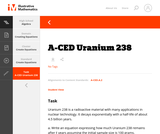Unrestricted Use
CC BY
Rating
0.0 stars

This is a task from the Illustrative Mathematics website that is one part of a complete illustration of the standard to which it is aligned. Each task has at least one solution and some commentary that addresses important aspects of the task and its potential use.

Subject:
Algebra
Material Type:
Activity/Lab
Provider:
Illustrative Mathematics
Provider Set:
Illustrative Mathematics
Author:
Illustrative Mathematics
08/06/2015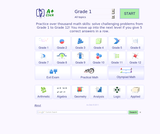Conditional Remix & Share Permitted
CC BY-NC-SA
Rating
4.0 stars

A+ Click is an interactive collection of more than 3700 math problems and answers for K-1 K-12 school program. It defines the personal level of math knowledge. You move up into the next level if you give 5 correct answers in a row. Practice makes perfect.

Subject:
Mathematics
Algebra
Geometry
Material Type:
Activity/Lab
Assessment
Game
Lecture Notes
Provider:
NSDL Staff
Provider Set:
Mathematics Gateways and Resources
Author:
Igor Kokcharov
02/16/2011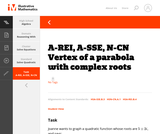Unrestricted Use
CC BY
Rating
0.0 stars

This is a task from the Illustrative Mathematics website that is one part of a complete illustration of the standard to which it is aligned. Each task has at least one solution and some commentary that addresses important aspects of the task and its potential use.

Subject:
Algebra
Material Type:
Activity/Lab
Provider:
Illustrative Mathematics
Provider Set:
Illustrative Mathematics
Author:
Illustrative Mathematics
08/06/2015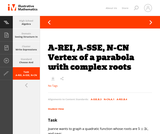Unrestricted Use
CC BY
Rating
0.0 stars

This is a task from the Illustrative Mathematics website that is one part of a complete illustration of the standard to which it is aligned. Each task has at least one solution and some commentary that addresses important aspects of the task and its potential use.

Subject:
Algebra
Material Type:
Activity/Lab
Provider:
Illustrative Mathematics
Provider Set:
Illustrative Mathematics
Author:
Illustrative Mathematics
08/06/2015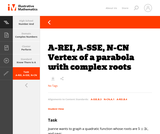Unrestricted Use
CC BY
Rating
0.0 stars

This is a task from the Illustrative Mathematics website that is one part of a complete illustration of the standard to which it is aligned. Each task has at least one solution and some commentary that addresses important aspects of the task and its potential use.

Subject:
Algebra
Material Type:
Activity/Lab
Provider:
Illustrative Mathematics
Provider Set:
Illustrative Mathematics
Author:
Illustrative Mathematics
08/06/2015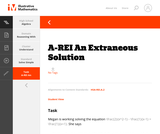Unrestricted Use
CC BY
Rating
0.0 stars

This is a task from the Illustrative Mathematics website that is one part of a complete illustration of the standard to which it is aligned. Each task has at least one solution and some commentary that addresses important aspects of the task and its potential use.

Subject:
Algebra
Material Type:
Activity/Lab
Provider:
Illustrative Mathematics
Provider Set:
Illustrative Mathematics
Author:
Illustrative Mathematics
08/06/2015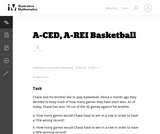Unrestricted Use
CC BY
Rating
0.0 stars

This task provides a simple but interesting and realistic context in which students are led to set up a rational equation (and a rational inequality) in one variable, and then solve that equation/inequality for an unknown variable.

Subject:
Mathematics
Algebra
Material Type:
Activity/Lab
Provider:
Illustrative Mathematics
Provider Set:
Illustrative Mathematics
Author:
Illustrative Mathematics
12/15/2012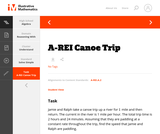Unrestricted Use
CC BY
Rating
0.0 stars

This is a task from the Illustrative Mathematics website that is one part of a complete illustration of the standard to which it is aligned. Each task has at least one solution and some commentary that addresses important aspects of the task and its potential use.

Subject:
Algebra
Material Type:
Activity/Lab
Provider:
Illustrative Mathematics
Provider Set:
Illustrative Mathematics
Author:
Illustrative Mathematics
08/06/2015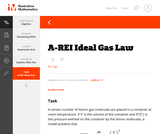Unrestricted Use
CC BY
Rating
0.0 stars

This is a task from the Illustrative Mathematics website that is one part of a complete illustration of the standard to which it is aligned. Each task has at least one solution and some commentary that addresses important aspects of the task and its potential use.

Subject:
Algebra
Material Type:
Activity/Lab
Provider:
Illustrative Mathematics
Provider Set:
Illustrative Mathematics
Author:
Illustrative Mathematics
08/06/2015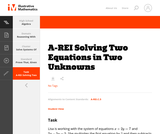Unrestricted Use
CC BY
Rating
0.0 stars

This is a task from the Illustrative Mathematics website that is one part of a complete illustration of the standard to which it is aligned. Each task has at least one solution and some commentary that addresses important aspects of the task and its potential use.

Subject:
Algebra
Material Type:
Activity/Lab
Provider:
Illustrative Mathematics
Provider Set:
Illustrative Mathematics
Author:
Illustrative Mathematics
08/06/2015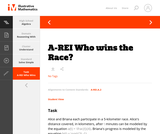Unrestricted Use
CC BY
Rating
0.0 stars

This is a task from the Illustrative Mathematics website that is one part of a complete illustration of the standard to which it is aligned. Each task has at least one solution and some commentary that addresses important aspects of the task and its potential use.

Subject:
Algebra
Material Type:
Activity/Lab
Provider:
Illustrative Mathematics
Provider Set:
Illustrative Mathematics
Author:
Illustrative Mathematics
08/06/2015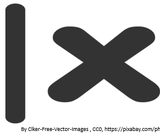Conditional Remix & Share Permitted
CC BY-NC
Rating
0.0 stars

In this seminar you will learn about the absolute value of numbers. You will learn how taking the absolute value affects both positive and negative numbers. You will use the techniques learned in this seminar to verify solutions to various other types of problems involving absolute value as you move forward. When looking at absolute value, you will identify how it can change a solution and the compare the difference that it makes in equations when there are multiple negative signs.StandardsCC.2.1.HS.F.2Apply properties of rational and irrational numbers to solve real world or mathematical problemsLearning TargetI can find the absolute value of a given number or numbers.Habits of MindPersistingCritical Thinking SkillAnalyze/evaluateAcademic/Concept VocabularyAbsolute valueNegativeNumber linePositive

Subject:
Mathematics
Algebra
Material Type:
Lesson Plan
Author:
Tracy Rains
03/19/2018Unrestricted Use
CC BY
Rating
0.0 stars

This module aims to acquaint you with the mathematical aspects of rings and groups and the underlying algebraic structures and when they are looked at as non-empty sets, how their elements are combined by binary operations as well as how those elements behave under transformations such finding inverses. Some non-empty sets, under the operation of addition or multiplication do not include the inverses of their elements as members of the set and they are called semi-groups. The non-empty sets that include the inverses of their elements are full fledged groups. This module fills the gap arising from basic mathematics.

Subject:
Algebra
Material Type:
Module
Provider:
African Virtual University
Provider Set:
OER@AVU
Author:
Othow Onak Giel
03/10/2018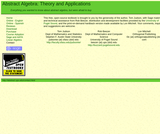Some Rights Reserved
Rating
0.0 stars

This text is intended for a one- or two-semester undergraduate course in abstract algebra. Traditionally, these courses have covered the theoretical aspects of groups, rings, and fields. However, with the development of computing in the last several decades, applications that involve abstract algebra and discrete mathematics have become increasingly important, and many science, engineering, and computer science students are now electing to minor in mathematics. Though theory still occupies a central role in the subject of abstract algebra and no student should go through such a course without a good notion of what a proof is, the importance of applications such as coding theory and cryptography has grown significantly.

Subject:
Algebra
Material Type:
Textbook
Provider:
University of Puget Sound
Author:
Thomas Judson
01/01/2016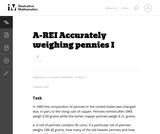Unrestricted Use
CC BY
Rating
4.0 stars

This problem involves solving a system of algebraic equations from a context: depending how the problem is interpreted, there may be one equation or two.

Subject:
Mathematics
Algebra
Material Type:
Activity/Lab
Provider:
Illustrative Mathematics
Provider Set:
Illustrative Mathematics
Author:
Illustrative Mathematics
08/15/2012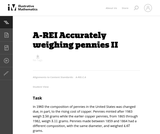Unrestricted Use
CC BY
Rating
0.0 stars

This task is a somewhat more complicated version of "Accurately weighing pennies I'' as a third equation is needed in order to solve part (a) explicitly. Instead, students have to combine the algebraic techniques with some additional problem-solving (numerical reasoning, informed guess-and-check, etc.)

Subject:
Mathematics
Algebra
Material Type:
Activity/Lab
Provider:
Illustrative Mathematics
Provider Set:
Illustrative Mathematics
Author:
Illustrative Mathematics
08/15/2012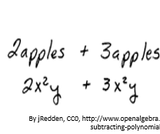Conditional Remix & Share Permitted
CC BY-NC
Rating
0.0 stars

It is important to understand polynomials and to be able to classify them based on the number of terms, as well as recognize the coefficients, and degrees. You must also understand how to perform mathematical operations on them. This seminar will focus on combining polynomials using addition and subtraction. It will be important to understand the rules to make sure you are combining only like terms. You will apply techniques you have learned involving exponents and general addition and subtraction rules. You will use the techniques learned in this seminar to verify solutions to various other types of polynomial problems as you move forward. When adding and subtracting polynomials, you will first identify the like terms to combine polynomials to their simplest form.StandardsCC.2.2.HS.D.3Extend the knowledge of arithmetic operations and apply to polynomials.

Subject:
Mathematics
Algebra
Material Type:
Lesson Plan
Author:
Tracy Rains
03/29/2018
RemixConditional Remix & Share Permitted
CC BY-NC
Rating
0.0 stars

Understanding adding and subtracting polynomials.StandardsCC.2.2.HS.D.3Extend the knowledge of arithmetic operations and apply to polynomials.

Subject:
Mathematics
Algebra
Material Type:
Homework/Assignment
Author:
Bailey Hauswirth
03/08/2021
RemixOnly Sharing Permitted
CC BY-ND
Rating
0.0 stars

It is important to understand polynomials and to be able to classify them based on the number of terms, as well as recognize the coefficients, and degrees. You must also understand how to perform mathematical operations on them. This seminar will focus on combining polynomials using addition and subtraction. It will be important to understand the rules to make sure you are combining only like terms. You will apply techniques you have learned involving exponents and general addition and subtraction rules. You will use the techniques learned in this seminar to verify solutions to various other types of polynomial problems as you move forward. When adding and subtracting polynomials, you will first identify the like terms to combine polynomials to their simplest form.StandardsCC.2.2.HS.D.3Extend the knowledge of arithmetic operations and apply to polynomials.

Subject:
Mathematics
Algebra
Material Type:
Activity/Lab
Lecture Notes
Author:
Maggie Elgert
07/23/2020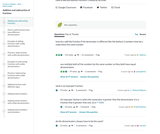Rating
0.0 stars

Kahn Academy video - How to add and subtract fractions with like and unlike denominators.

Subject:
Education
Mathematics
Algebra
Material Type:
Interactive
Teaching/Learning Strategy
Provider:
Florida Center for Research in Science, Technology, Engineering, and Mathematics
Provider Set:
iCPALMS: A Standards-based K-12 Resources and Tools Pathway
Author:
Salman Khan
03/28/2011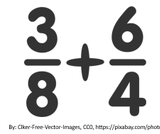Conditional Remix & Share Permitted
CC BY-NC
Rating
0.0 stars

In this seminar you will continue your learning of rational expressions adding and subtracting them as stand alone problems, as well as in real-world situations  You will continue to use strategies for simplifying complex situations into more solvable situations.StandardsCCSI: HS: Algebra: HSA-SSE.A. Interpret the structure of expressions.CCSI: HS: Algebra: HSA-SSE.B. Write expressions in equivalent forms to solve problems.

Subject:
Mathematics
Algebra
Material Type:
Lesson Plan
Author:
Tracy Rains
04/03/2018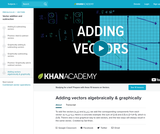Unrestricted Use
CC BY
Rating
0.0 stars

To add the vectors (x₁,y₁) and (x₂,y₂), we add the corresponding components from each vector: (x₁+x₂,y₁+y₂). Here's a concrete example: the sum of (2,4) and (1,5) is (2+1,4+5), which is (3,9). There's also a nice graphical way to add vectors, and the two ways will always result in the same vector.​​

Subject:
Algebra
Material Type:
Lesson
Provider:
Provider Set:
Author:
Salman Khan
02/07/2018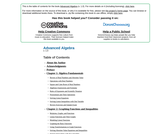Conditional Remix & Share Permitted
CC BY-NC-SA
Rating
0.0 stars

Intermediate Algebra is the second part of a two-part course in Algebra. Written in a clear and concise manner, it carefully builds on the basics learned in Elementary Algebra and introduces the more advanced topics required for further study of applications found in most disciplines. Used as a standalone textbook, it offers plenty of review as well as something new to engage the student in each chapter. Written as a blend of the traditional and graphical approaches to the subject, this textbook introduces functions early and stresses the geometry behind the algebra. While CAS independent, a standard scientific calculator will be required and further research using technology is encouraged.

Subject:
Algebra
Material Type:
Textbook
Author:
John Redden
11/18/2019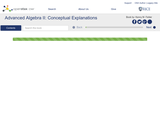Unrestricted Use
CC BY
Rating
0.0 stars

This is the Conceptual Explanations part of Kenny Felder's course in Advanced Algebra II. It is intended for students to read on their own to refresh or clarify what they learned in class. This text is designed for use with the "Advanced Algebra II: Homework and Activities" and the "Advanced Algebra II: Teacher's Guide" collections (coming soon) to make up the entire course.

Subject:
Algebra
Material Type:
Module
Provider:
Rice University
Provider Set:
OpenStax CNX
Author:
Kenny Felder
02/21/2014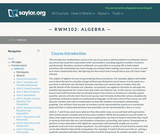Unrestricted Use
CC BY
Rating
0.0 stars

This course discusses how to use algebra for a variety of everyday tasks, such as calculate change without specifying how much money is to be spent on a purchase, analyzing relationships by graphing, and describing real-world situations in business, accounting, and science.

Subject:
Algebra
Material Type:
Full Course
Provider:
The Saylor Foundation
08/28/2013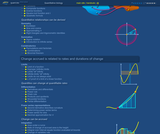Conditional Remix & Share Permitted
CC BY-SA
Rating
0.0 stars

In this video, we explore some concepts fundamental to algebra. To streamline the discussion of relationships between physical quantities, we introduce variables, functions, composition, and inverse. By thinking about the concept of an inverse function, we obtain our first glimpse of the imaginary root (i.e. square-root of -1) and the complex plane.

Subject:
Mathematics
Algebra
Material Type:
Lecture Notes
Provider:
Look At Physics
Provider Set:
A Mathematical Way to Think About Biology
Author:
David Liao
10/08/2011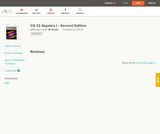Conditional Remix & Share Permitted
CC BY-NC-SA
Rating
2.0 stars

A work in progress, CK-12's Algebra I Second Edition is a clear presentation of algebra for the high school student. Topics include: Equations and Functions, Real Numbers, Equations of Lines, Solving Systems of Equations and Quadratic Equations.

Subject:
Algebra
Functions
Material Type:
Textbook
Provider:
CK-12 Foundation
Provider Set:
CK-12 FlexBook
Author:
Andrew
Annamaria
Anne
Eve
Farbizio
Gloag
Rawley
12/03/2010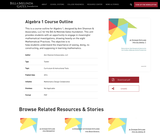Rating
0.0 stars

Algebra 1 Course Outline

Subject:
Algebra
Material Type:
Textbook
10/03/2017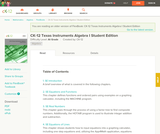Conditional Remix & Share Permitted
CC BY-NC-SA
Rating
0.0 stars

CK-12's Texas Instruments Algebra I Student Edition Flexbook allows students to better utilize a graphing calculator in understanding the fundamental concepts of algebra.

Subject:
Algebra
Material Type:
Textbook
Provider:
CK-12 Foundation
Provider Set:
CK-12 FlexBook
Author:
Jordan, Lori
12/08/2010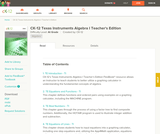Conditional Remix & Share Permitted
CC BY-NC-SA
Rating
0.0 stars

CK-12's Texas Instruments Algebra I Teacher's Edition Flexbook allows an Instructor to teach students to better utilize a graphing calculator in understanding the fundamental concepts of algebra.

Subject:
Algebra
Material Type:
Textbook
Provider:
CK-12 Foundation
Provider Set:
CK-12 FlexBook
Author:
Jordan, Lori
12/13/2010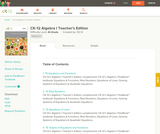Conditional Remix & Share Permitted
CC BY-NC-SA
Rating
0.0 stars

CK-12 Foundation's Algebra I Teacher's Edition FlexBook complements CK-12's Algebra I Student Edition. The solution and assessment guides are available upon request.

Subject:
Algebra
Material Type:
Textbook
Provider:
CK-12 Foundation
Provider Set:
CK-12 FlexBook
Author:
Fay-Zenk, Mary
McFarland, Andrew
11/06/2009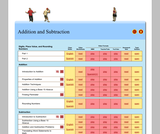Conditional Remix & Share Permitted
CC BY-NC-SA
Rating
0.0 stars

Professor Perez and his favorite student Charlie have the tools that can help you. We have five primary types of study materials: class notes, video worksheets, video lectures, practice problems, and practice quizzes.

Subject:
Algebra
Numbers and Operations
Material Type:
Homework/Assignment
Lecture
Lecture Notes
Provider:
Provider Set:
Individual Authors
Author:
Candice Harrington
Larry Perez
Patrick Quigley
04/19/2013Conditional Remix & Share Permitted
CC BY-NC-SA
Rating
0.0 stars

This site teaches Arithmetic with Polynomials and Rational Expressions to High Schoolers through a series of 4333 questions and interactive activities aligned to 26 Common Core mathematics skills.

Subject:
Mathematics
Algebra
Material Type:
Activity/Lab
Interactive
Provider:
Provider Set:
01/09/2015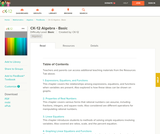Conditional Remix & Share Permitted
CC BY-NC-SA
Rating
0.0 stars

CK-12 Foundation's Basic Algebra FlexBook is an introduction to the algebraic topics of functions, equations, and graphs for middle-school and high-school students.

Subject:
Algebra
Functions
Material Type:
Textbook
Provider:
CK-12 Foundation
Provider Set:
CK-12 FlexBook
Author:
Farbizio, Annamaria
Gloag, Andrew
Gloag, Anne
Kramer, Melissa